# Fractions Percents Expressing Percents as Fractions and Fractions

• Slides: 12Fractions & Percents Expressing Percents as Fractions and Fractions as PercentsPercents n A percent is a part to whole ratio that compares a number to 100. n Percent comes from the Latin word centum, which means “hundred”. n To write a percent as a fraction, express the ratio as a fraction with a denominator of 100. n 80% means 80 out of 100 or 80/100.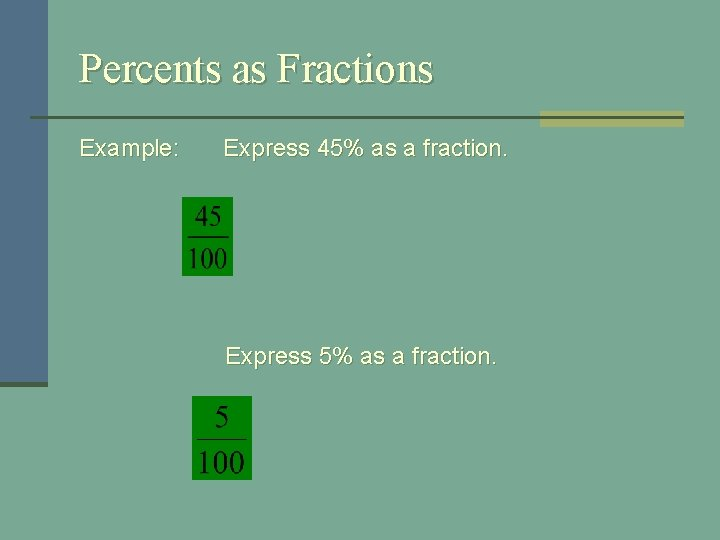Percents as Fractions Example: Express 45% as a fraction. Express 5% as a fraction.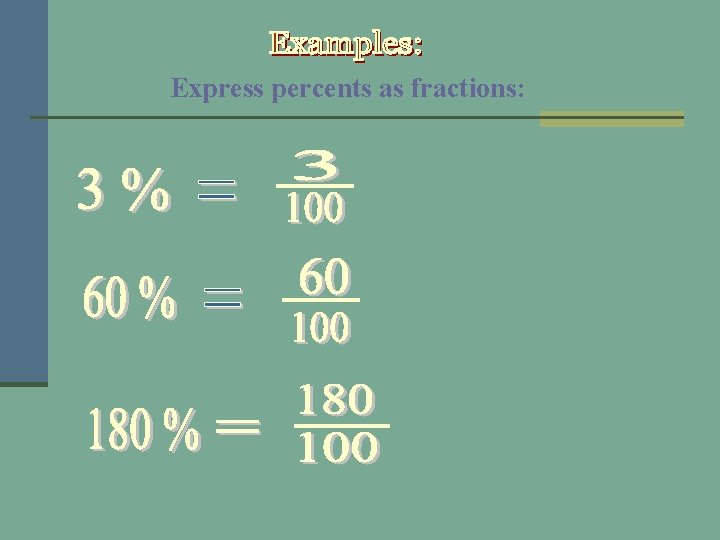Express percents as fractions:Your Turn Express Percents as Fractions 1. 45% 2. 7% 3. 223%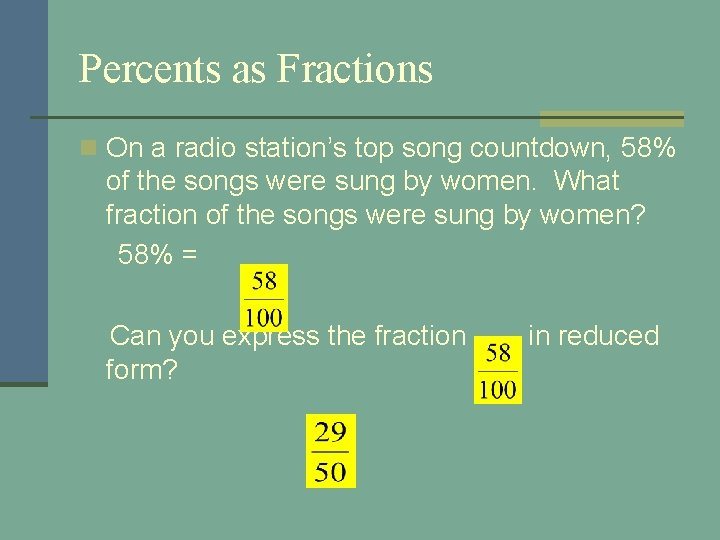Percents as Fractions n On a radio station’s top song countdown, 58% of the songs were sung by women. What fraction of the songs were sung by women? 58% = Can you express the fraction form? in reduced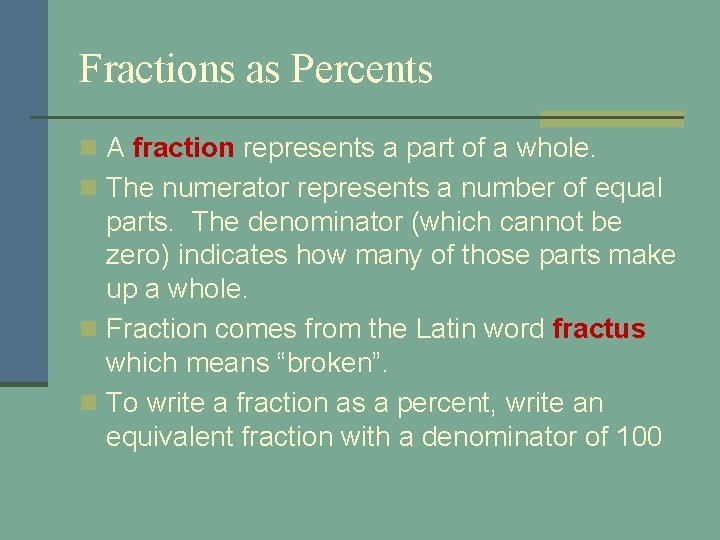Fractions as Percents n A fraction represents a part of a whole. n The numerator represents a number of equal parts. The denominator (which cannot be zero) indicates how many of those parts make up a whole. n Fraction comes from the Latin word fractus which means “broken”. n To write a fraction as a percent, write an equivalent fraction with a denominator of 100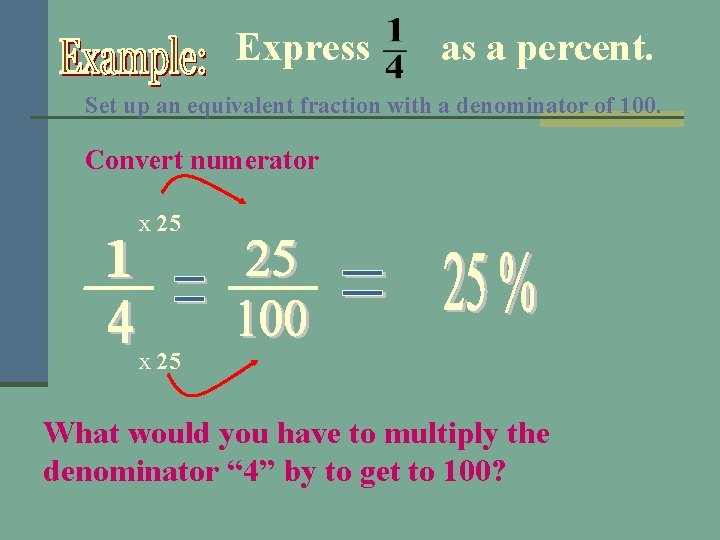Express as a percent. Set up an equivalent fraction with a denominator of 100. Convert numerator x 25 What would you have to multiply the denominator “ 4” by to get to 100?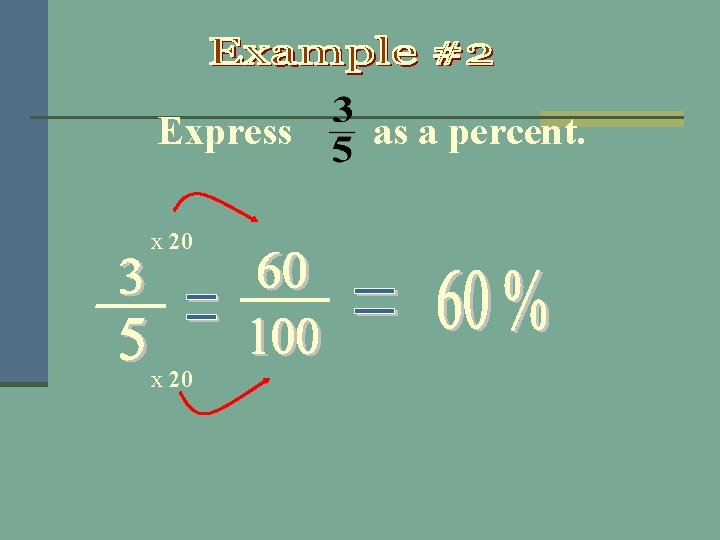Express x 20 as a percent.Fractions as Percents Your Turn 1. 80% 2. 5% 3. 16% 4. 90%Fractions as Percents n You buy a shirt that is cotton, n polyester, and the rest is linen. a) What percent of the shirt is cotton? n b) What percent of the shirt is polyester? n c) What percent of the shirt is linen? n a) b) c) 25% 60% 15%QUESTIONS?